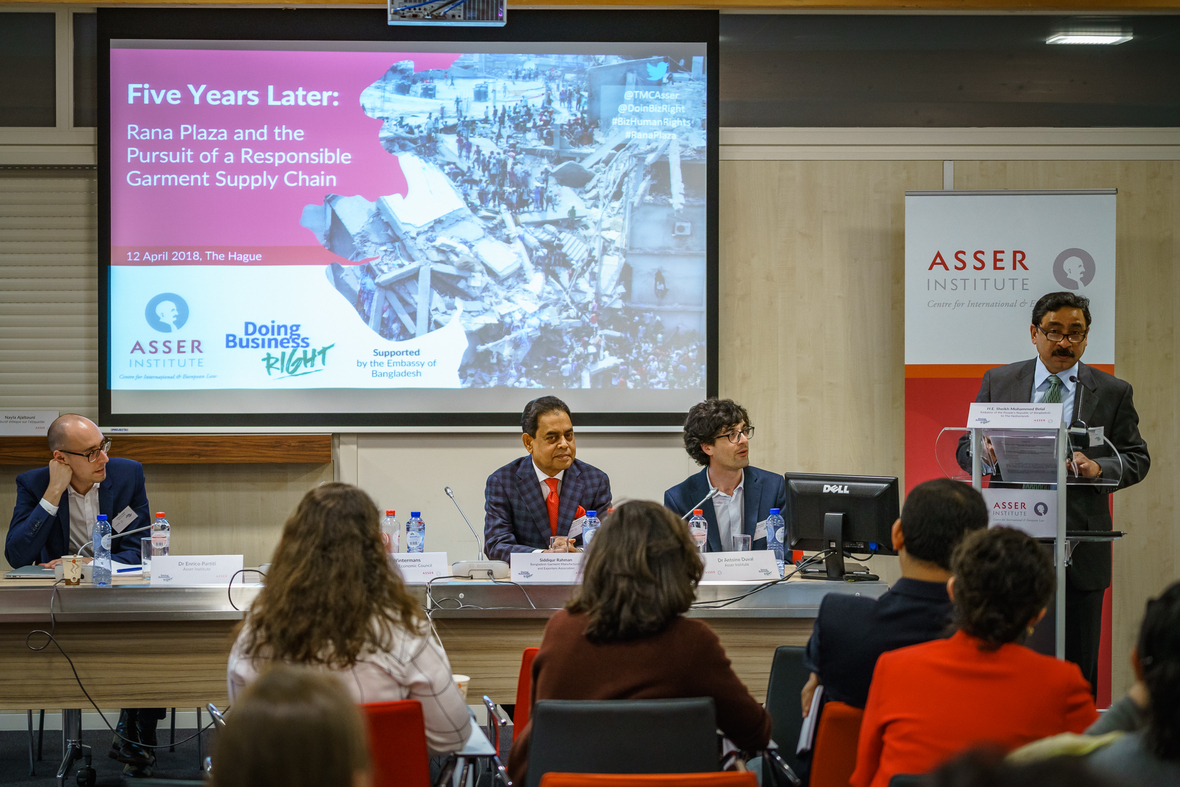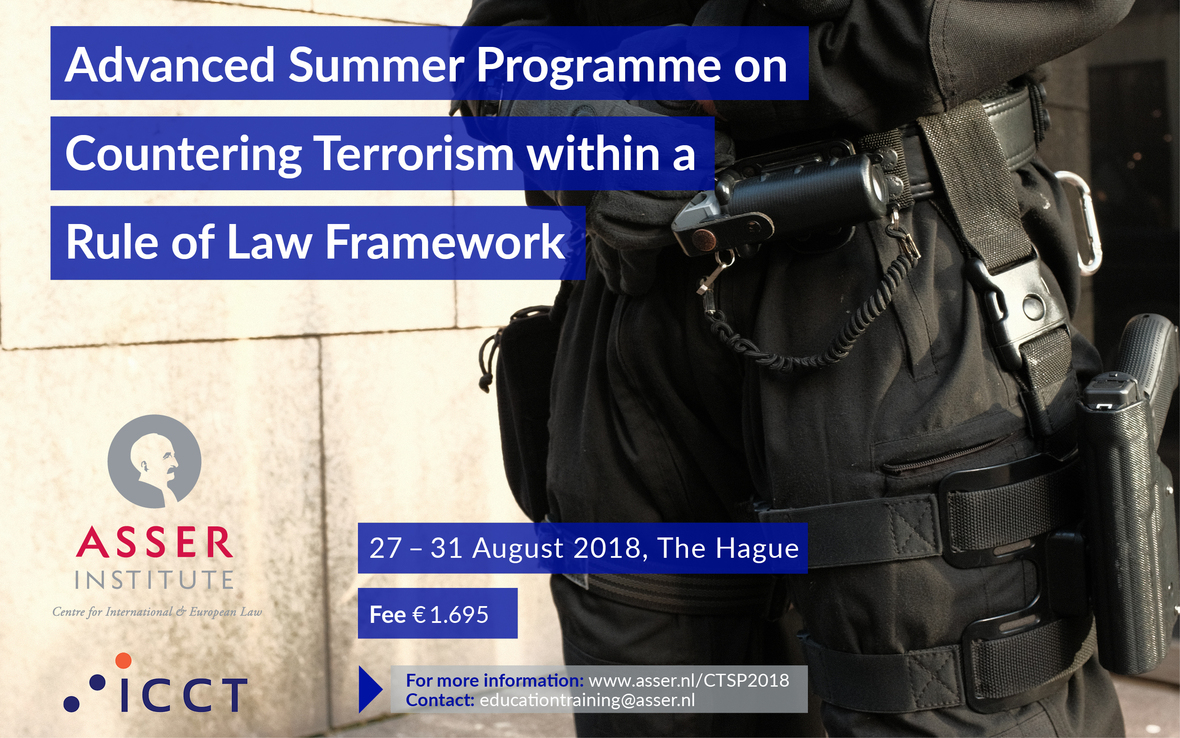Like   Tweet   in

## Asser Today May 2018

 table div table+table+table div table{width:100%;padding:0}table div table+table+table div table img{width:96.23%;padding:0;float:none}table div table+table+table div table td{width:100%;padding:0 1.88% 18px}/* styles */# Asser News

## Asser-Verfassungsblog Joint Online Symposium: Courts and Counter-Terrorism

The Asser Institute and Verfassungsblog launched their second joint online symposium titled ‘Courts and Counter-Terrorism’. The symposium featured 9 blog posts edited by Asser’s senior researcher Dr. Christophe Paulussen who also wrote the introductary blogpost “Courts and Counter-Terrorism: the Last Line of Defence?”. The symposium analyses important judgments and legislative developments in several countries, namely the Netherlands, the United Kingdom, Finland, Italy, Turkey, China, France, Germany and Spain. Asser researchers Dr. Bérénice Boutin and Dr. Rumyana Grozdanova also participated in the symposium. Read more here.

## Other Newstable div table+table+table+table+table+table+table+table+table div table,table.module-8{width:50.38%;float:left;padding:0}table div table+table+table+table+table+table+table+table+table div table a{border:0 none;text-decoration:none}table div table+table+table+table+table+table+table+table+table div table img{width:100%!important;border:0 none;text-decoration:none}table div table+table+table+table+table+table+table+table+table div table td{width:100%;padding:0 20px 20px 0}/* styles */
 table div table+table+table+table+table+table+table+table+table+table div table,table.module-9{width:49.62%;float:left;padding:0}table div table+table+table+table+table+table+table+table+table+table div table a{border:0 none;text-decoration:none}table div table+table+table+table+table+table+table+table+table+table div table img{width:100%!important;border:0 none;text-decoration:none}table div table+table+table+table+table+table+table+table+table+table div table td{width:100%;padding:0 20px 20px 0}/* styles *//* styles */

## Selected Publications

▪ Ernst Hirsch Ballin, ‘Learning democracy: Points of reference for a peaceful counteroffensive’. T.M.C. Asser Institute Research paper, May 2018.
 ▪ Ernst Hirsch Ballin, ‘Learning democracy: Points of reference for a peaceful counteroffensive’. T.M.C. Asser Institute Research paper, May 2018.

▪ Sofia Stolk, ‘Imagining scenes of mass atrocity from afar: maps and landscapes at the International Criminal Court’ in London Review of International Law, Volume 5, Issue 3, 1 November 2017, Pages 425–451. Published: 24 May 2018
 ▪ Sofia Stolk, ‘Imagining scenes of mass atrocity from afar: maps and landscapes at the International Criminal Court’ in London Review of International Law, Volume 5, Issue 3, 1 November 2017, Pages 425–451. Published: 24 May 2018

▪ Antoine Duval and Catherine Dunmore, ‘The Case for a Court of Arbitration for Business and Human Rights’. T.M.C. Asser Institute Policy Brief., May 2018. *
 ▪ Antoine Duval and Catherine Dunmore, ‘The Case for a Court of Arbitration for Business and Human Rights’. T.M.C. Asser Institute Policy Brief., May 2018. *
 /* styles */ Asser research papers and policy briefs can be downloaded on SSRN.
 table div table+table+table+table+table+table+table+table+table+table+table+table+table+table+table div table{width:100%;padding:0}table div table+table+table+table+table+table+table+table+table+table+table+table+table+table+table div table img{width:96.23%;padding:0;float:none}table div table+table+table+table+table+table+table+table+table+table+table+table+table+table+table div table td{width:100%;padding:0 1.88% 18px}/* styles */# Upcoming Events and Education

The Asser Institute has extensive experience in delivering postgraduate training programmes and in knowledge dissemination in the field of international and European law.

 table div table+table+table+table+table+table+table+table+table+table+table+table+table+table+table+table+table div table{width:100%;padding:0}table div table+table+table+table+table+table+table+table+table+table+table+table+table+table+table+table+table div table img{width:96.23%;padding:0;float:none}table div table+table+table+table+table+table+table+table+table+table+table+table+table+table+table+table+table div table td{width:100%;padding:0 1.88% 18px}/* styles */table div table+table+table+table+table+table+table+table+table+table+table+table+table+table+table+table+table+table div table,table.module-17{width:43.21%;float:right;padding:0}table div table+table+table+table+table+table+table+table+table+table+table+table+table+table+table+table+table+table div table a{border:0 none;text-decoration:none}table div table+table+table+table+table+table+table+table+table+table+table+table+table+table+table+table+table+table div table img{width:100%!important;border:0 none;text-decoration:none}table div table+table+table+table+table+table+table+table+table+table+table+table+table+table+table+table+table+table div table td{width:100%;padding:0 0 20px 20px}/* styles */ 4 June Launch of the book “The Prevention of Gross Human Rights Violations Under International Human Rights Law”, followed by a panel discussion Over the past decades, there has been a great deal of attention for concepts aiming to prevent gross human rights violations, such as conflict prevention and the responsibility to protect. Despite this shift in attention towards prevention, it has remained unclear what legal obligations states have to prevent gross human rights violations under international human rights law. For example, it is unclear what types of obligations states have at different points in time, when they are triggered, what concrete measures they may require and how they apply outside a state’s territory. This book launch of 'The Prevention of Gross Human Rights Violations Under International Human Rights Law' will offer a structured exploration of the topic and discuss some of the main findings. More information and registration
 table div table+table+table+table+table+table+table+table+table+table+table+table+table+table+table+table+table+table+table div table,table.module-18{width:56.79%;float:left;padding:0}table div table+table+table+table+table+table+table+table+table+table+table+table+table+table+table+table+table+table+table div table a{border:0 none;text-decoration:none}table div table+table+table+table+table+table+table+table+table+table+table+table+table+table+table+table+table+table+table div table img{width:100%!important;border:0 none;text-decoration:none}table div table+table+table+table+table+table+table+table+table+table+table+table+table+table+table+table+table+table+table div table td{width:100%;padding:0 20px 20px 0}/* styles */ 15 May From Strasbourg to Hamburg: the Human Dimension of the Law of the Sea This event deals with legal issues and challenges concerning the protection of individuals at sea. The topic is analysed and discussed from different institutional angles, notably the ECtHR and the ITLOS, and by considering the interaction and complementarity of different legal frameworks, such as the law of sea, human rights law and refugee law. This event marks the beginning of the new cooperation between the Asser Institute and the Law Faculty of the University of Basel aimed at addressing the ‘Human Dimension of the Law of the Sea’. More information and registration09 - 13 July International Lawyering in a Public Interest Summer Programme

What does it take to practice international law for public interests? This summer programme will explore the challenges of public interest advocacy in international law, from questions of practice to finding or starting a firm, and from finding funding to identifying causes for advocacy. We will also raise critical questions, to challenge what it means to practice public interest advocacy in the international system. Our group of lecturers includes leaders in practice, including advocates from major firms and pro-bono start-ups, as well as NGOs, the diplomatic community, and academia.27 - 31 August Advanced Summer Programme on Countering Terrorism within a Rule of Law Framework

This Advanced Summer Programme offers an in-depth look at the challenges that come with adopting and implementing counter-terrorism measures while ensuring respect for human rights, fundamental freedoms and the rule of law. During an intensive week, experts from academia and practice will explore (international) legal aspects of counter-terrorism and provide key insights into current issues and best practices.

The programme covers a wide range of topics (including the definition of terrorism in international and domestic law, legal limits to military responses to terrorism, criminal justice responses to terrorism, evidentiary issues and other prosecutorial challenges, administrative and security measures), and comports various types of activities (including lectures, interactive sessions, study visits, group discussions, panel presentations).

 table div table+table+table+table+table+table+table+table+table+table+table+table+table+table+table+table+table+table+table+table+table+table+table div table{width:100%;padding:0}table div table+table+table+table+table+table+table+table+table+table+table+table+table+table+table+table+table+table+table+table+table+table+table div table img{width:96.23%;padding:0;float:none}table div table+table+table+table+table+table+table+table+table+table+table+table+table+table+table+table+table+table+table+table+table+table+table div table td{width:100%;padding:0 1.88% 18px}/* styles */table.module-23{width:43.21%;padding:0}table div table+table+table+table+table+table+table+table+table+table+table+table+table+table+table+table+table+table+table+table+table+table+table+table div table{width:43.21%;float:none;margin-left:auto;margin-right:auto;padding:0}table div table+table+table+table+table+table+table+table+table+table+table+table+table+table+table+table+table+table+table+table+table+table+table+table div table a{border:0 none;text-decoration:none}table div table+table+table+table+table+table+table+table+table+table+table+table+table+table+table+table+table+table+table+table+table+table+table+table div table img{width:100%!important;border:0 none;text-decoration:none}table div table+table+table+table+table+table+table+table+table+table+table+table+table+table+table+table+table+table+table+table+table+table+table+table div table td{width:100%;padding:0}/* styles */
 table div table+table+table+table+table+table+table+table+table+table+table+table+table+table+table+table+table+table+table+table+table+table+table+table+table div table,table.module-24{width:43.96%;float:left;padding:0}table div table+table+table+table+table+table+table+table+table+table+table+table+table+table+table+table+table+table+table+table+table+table+table+table+table div table a{border:0 none;text-decoration:none}table div table+table+table+table+table+table+table+table+table+table+table+table+table+table+table+table+table+table+table+table+table+table+table+table+table div table img{width:100%!important;border:0 none;text-decoration:none}table div table+table+table+table+table+table+table+table+table+table+table+table+table+table+table+table+table+table+table+table+table+table+table+table+table div table td{width:100%;padding:0 20px 20px 0}/* styles */ Yearbook of International Sports Arbitration 2016 - Volume 2 - Yearbook of International Sports Arbitration Print ISBN 978-94-6265-236-1 EBook/Online ISBN: 978-94-6265-237-8 Distributed for T.M.C. Asser Press by Springer Read this book on SpringerLink Erika Hasler’s article ‘Back to the Future: The First CAS Arbitrators on CAS’s First Award and its Evolution Since Then’, published in the Yearbook of International Sports Arbitration 2016 (TMC Asser Press) is available on SpringerLink open access for four weeks only.
 /* styles */
 table div table+table+table+table+table+table+table+table+table+table+table+table+table+table+table+table+table+table+table+table+table+table+table+table+table+table+table div table{width:100%;padding:0}table div table+table+table+table+table+table+table+table+table+table+table+table+table+table+table+table+table+table+table+table+table+table+table+table+table+table+table div table img{width:96.23%;padding:0;float:none}table div table+table+table+table+table+table+table+table+table+table+table+table+table+table+table+table+table+table+table+table+table+table+table+table+table+table+table div table td{width:100%;padding:0 1.88% 18px}/* styles */# Newsletter of the T.M.C. Asser Instituut

Editor:
Faten Bushehri

The T.M.C. Asser Instituut carries out research on developments in international and European law and its potential for serving the cultivation of trust and respect in the global, regional, national and local societies in which the law operates.

 table div table+table+table+table+table+table+table+table+table+table+table+table+table+table+table+table+table+table+table+table+table+table+table+table+table+table+table+table+table div table{width:100%;padding:0}table div table+table+table+table+table+table+table+table+table+table+table+table+table+table+table+table+table+table+table+table+table+table+table+table+table+table+table+table+table div table img{width:96.23%;padding:0;float:none}table div table+table+table+table+table+table+table+table+table+table+table+table+table+table+table+table+table+table+table+table+table+table+table+table+table+table+table+table+table div table td{width:100%;padding:0 1.88% 18px}/* styles */